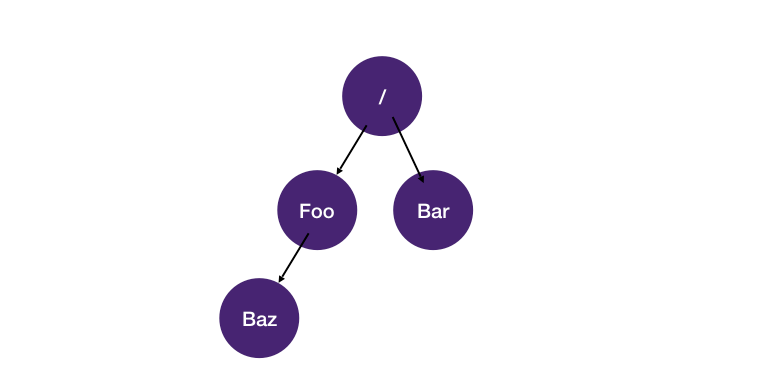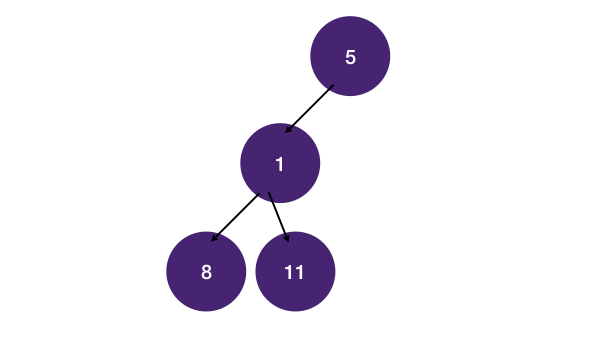# DFS on Trees

Prereq: DFS Introduction

## Think like a node

The key to solving tree problems using DFS is to think from the perspective of a node instead of looking at the whole tree. This is in line with how recursion is written. Reason from a node's perspective, decide how the current node should be proceeded, then recurse on children and let recursion takes care of the rest.

When you are a node, the only things you know are 1) your value and 2) how to get to your children. So the recursive function you write manipulates these things.

The template for DFS on tree is:

``````1function dfs(node, state):
2    if node is null:
3        ...
4        return
5
6    left = dfs(node.left, state)
7    right = dfs(node.right, state)
8
9        ...
10
11    return ...``````

## Defining the recursive function

Two things we need to decide to define the function:

### 1. Return value (passing value up from child to parent)

What do we want to return after visiting a node. For example, for the max depth problem this is the max depth for the current node's subtree. If we are looking for a node in the tree, we'd want to return that node if found, else return null. Use the return value to pass information from children to parent.

### 2. Identify state(s) (passing value down from parent to child)

What states do we need to maintain to compute the return value for the current node. For example, to know if the current node's value is larger than its parent we have to maintain the parent's value as a state. State becomes DFS's function arguments. Use states to pass information from parent to children.

Consider the problem of pretty-print a binary tree. Given directory treeWe want to "pretty-print" the directory structure with indents like this:

``````1/
2  foo
3    baz
4  bar``````

We can pass the current indent level as a state of the recursive call.

``````1indent_per_level = '  '
2function dfs(node, indent_level):
3  ...
4  current_indent_level = indent_level + indent_per_level
5  print(current_indent_level + node.val)
6  dfs(node, current_indent_level)``````

## Using return value vs. global variable

Consider the problem of finding the maximum value in a binary tree.`11` is the large value.

### Using return value (divide and conquer)

One way to solve it is to use return value to pass the maximum value we have encountered back to parent node, and let the parent node compare it with the return value from the other child. This is more of a divide and conquer approach. We have seen this in merge sort.

``````1function dfs(node):
2  if node is null:
3    return MIN_VALUE
4
5  left_max_val = dfs(node.left)
6  right_max_val = dfs(node.right)
7  return max(node.val, left_max_val, right_max_val)``````

### Using global variable

Another way to solve it is to traverse the tree while keeping a global variable that keeps track of the maximum value we have encountered. After the dfs, we return the global variable.

The recursive function `dfs` does not return any value in this case. We "fire-and-forget" the `dfs` call.

``````1...
2# global variable to record current max value
3# initialize to minimum value possible so any node will be larger
4max_val = MIN_VALUE
5
6function dfs(node):
7  if node is null:
8    return
9
10  if node.val > max_val: # update the global variable if current value is larger
11    max_val = node.val
12
13  # recurse
14  dfs(node.left)
15  dfs(node.right)
16
17function get_max_val(root)
18  dfs(root) # kick off dfs from root node
19  return max_val``````

It's more of a personal preference which one you use. One could argue global variables are bad and therefore the divide and conquer style is better. However, sometimes it's easier to use a global variable. Recall that divide and conquer has two steps - partition and merge. If the merge step is complex, then using a global variable might simplify things.

Still not clear? Submit the part you don't understand to our editors.